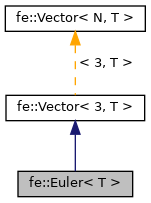Free Electron
fe::Euler< T > Class Template Reference

Special vector for concatenated Euler angles. More...

#include <Euler.h>

Inheritance diagram for fe::Euler< T >:[legend]

## Public Member Functions

Euler (T x, T y, T z)

Euler (const Vector< 3, T > &other)

Euler (const Euler< T > &other)

Euler (const Quaternion< T > &quaternion)

Euler (const Matrix< 3, 4, T > &matrix)

Euler< T > & operator= (const Euler< T > &euler)

Euler< T > & operator= (const Quaternion< T > &quaternion)

Euler< T > & operator= (const Matrix< 3, 4, T > &matrix)

operator Matrix< 3, 4, T > (void) constPublic Member Functions inherited from fe::Vector< 3, T >
Vector (const Vector< N, T > &other)

Vector (const Vector< N, U > &other)

Vector (const Vector< N+1, U > &other)

Vector (const Vector< N+2, U > &other)

Vector (const Vector< N-1, U > &other)

Vector (const Vector< N-2, U > &other)

Vector (const T *array)

Vector (T x, T y, T z, T w, T v, T u)

Vector (T x, T y, T z, T w, T v)

Vector (T x, T y, T z, T w)

Vector (T x, T y, T z)

Vector (T x, T y)

Vector (T x)

Vector (const Vector< 2, U > &other)

const T & operator[] (unsigned int index) const

T & operator[] (unsigned int index)

T * raw (void)

const T * raw (void) const

const T * temp (void) const

Vector< N, T > & operator= (const T *array)

Vector< N, T > & operator= (const Vector< N, T > &other)

Vector< N, T > & operator= (const Vector< N, U > &other)

Vector< N, T > & operator= (const Vector< N+1, T > &other)

Vector< N, T > & operator= (const Vector< N+1, U > &other)

Vector< N, T > & operator= (const Vector< N+2, U > &other)

Vector< N, T > & operator= (const Vector< N-1, U > &other)

Vector< N, T > & operator= (const Vector< N-2, U > &other)

bool operator== (const Vector< N, T > &other)

bool operator!= (const Vector< N, T > &other)Public Attributes inherited from fe::Vector< 3, T >
m_data [N]

## Detailed Description

### template<typename T> class fe::Euler< T >

Special vector for concatenated Euler angles.

This represents a multiplication of three rotation matrices, about X, Y, and Z, in that order.

These are raw post-multiplication steps, not the pre-multiplication concatenation convention like Matrix::rotate, so the conversion to matrix rotates in the reverse order (rotate Z, rotate Y, then rotate X).

In a X-forward Z-up world this maps into a transformation of heading CCW, pitch down, and then bank right.

The documentation for this class was generated from the following file: